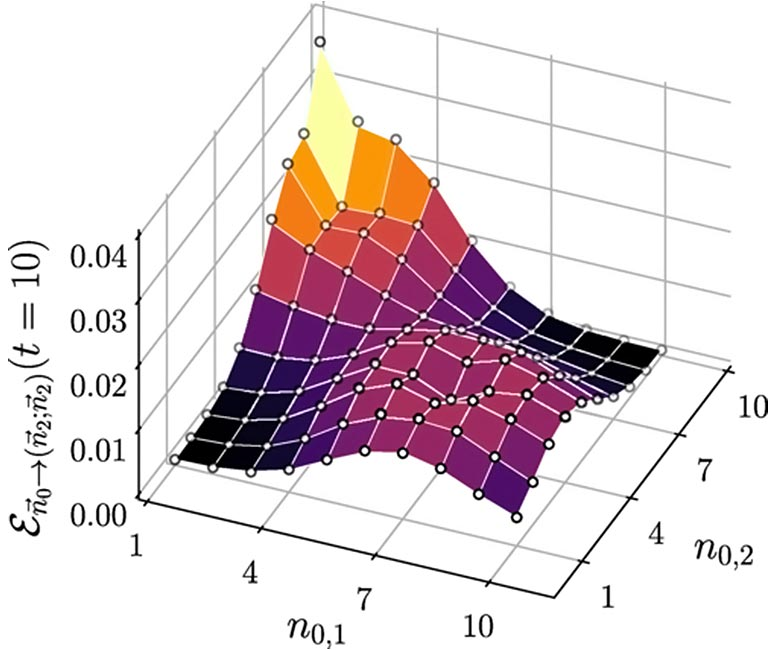# 100-Year-Old Physics Problem Finally Solved – Accurately Predicts Transmission of Infectious DiseasesA Bristol academic has achieved a milestone in statistical/mathematical physics by solving a 100-year-old physics problem – the discrete diffusion equation in finite space.

The long-sought-after solution could be used to accurately predict encounter and transmission probability between individuals in a closed environment, without the need for time-consuming computer simulations.

In his paper, published in Physical Review X, Dr. Luca Giuggioli from the Department of Engineering Mathematics at the University of Bristol describes how to analytically calculate the probability of occupation (in discrete time and discrete space) of a diffusing particle or entity in a confined space – something that until now was only possible computationally.

Dr. Giuggioli said: “The diffusion equation models random movement and is one of the fundamental equations of physics.  The analytic solution of the diffusion equation in finite domains, when time and space is continuous, has been known for a long time.

“However, to compare model predictions with empirical observations, one needs to study the diffusion equation in finite space. Despite the work of illustrious scientists such as Smoluchowski, Pólya, and other investigators of yore, this has remained an outstanding problem for over a century—until now.

“Excitingly, the discovery of this exact analytic solution allows us to tackle problems that were almost impossible in the past because of the prohibitive computational costs.”

The finding has far-reaching implications across a range of disciplines and possible applications include predicting molecules diffusing inside cells, bacteria roaming in a petri dish, animals foraging within their home ranges, or robots searching in a disaster area.

It could even be used to predict how a pathogen is transmitted in a crowd between individuals.

Solving the conundrum involved the joint use of two techniques: special mathematical functions known as Chebyshev polynomials, and a technique invented to tackle electrostatic problems, the so-called method of images.

This approach allowed Dr. Giuggioli to construct hierarchically the solution to the discrete diffusion equation in higher dimension from the one in lower dimensions.

Reference: “Exact Spatiotemporal Dynamics of Confined Lattice Random Walks in Arbitrary Dimensions: A Century after Smoluchowski and Pólya” by Luca Giuggioli, 28 May 2020, Physical Review X.
DOI: 10.1103/PhysRevX.10.021045